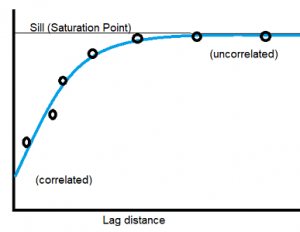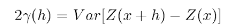# Variogram: Definition, Examples

Share onA variogram plots differences in pairs of data points, separated by a lag distance. If a process has a strong spatial correlation, the variogram function will be increasing, usually reaching a saturation point. If the function shows steady behavior, that indicates an absence of spatial correlation.

A variogram is an effective tool for describing the behavior of non-stationary, spatial random processes. It is used primarily in spatial statistics, geostatistics, and statistical design; In geostatistics, it is an “essential step” for analyzing spatial variability (Gómez-Hernández et al., 1999).

## The Variogram Function

The variogram measures dissimilarity in a relatively simple way. Pairs of data points are separated by a specified lag distance. Lags are chosen by the user, and depend on the type of data you’re dealing with. For example, lags might be calculated for data points that are 1 km apart, then 2 km apart, then 3 km apart. Or they might be calculated for points 5, 10, and 15 cm apart. A lag tolerance is set, so that values that aren’t exactly the specified distance fall into a specified lag. This is usually in between lags. For example, a distance of 7 cm rounds down to the 5 cm lag, and a 9 cm lag rounds up to a 10 cm lag (note: if you’re familiar with histogram bins, lag tolerances work in much the same way).

These lags are analyzed for their mean squared differences. The degree of dissimilarity between the Z(x) and Z(x + h) functions are defined with the variogram function γ(h):Unlike the covariance function, you don’t need to know the mean. Plus, it can handle means that aren’t constant.

## References

Gómez-Hernández et al.(1999). geoENV II — Geostatistics for Environmental Applications. Springer Science & Business Media.
Orshansky, M. et al. (2007). Design for Manufacturability and Statistical Design: A Constructive Approach. Springer Science & Business Media.

CITE THIS AS:
Stephanie Glen. "Variogram: Definition, Examples" From StatisticsHowTo.com: Elementary Statistics for the rest of us! https://www.statisticshowto.com/variogram/
---------------------------------------------------------------------------Need help with a homework or test question? With Chegg Study, you can get step-by-step solutions to your questions from an expert in the field. Your first 30 minutes with a Chegg tutor is free!